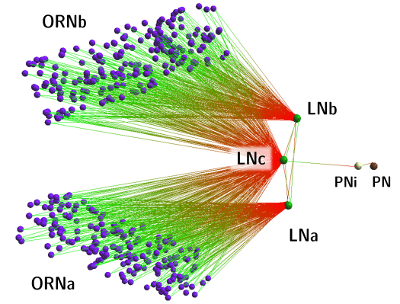# CNrun example

Supplementary material for the PLoS paper “Competition-based model of pheromone component ratio detection in the moth.” by Zavada A et al.

Note: the experimental setup described here uses CNrun 1.x, not the newer, Lua-powered version 2 (for that, check out this annotated script).

## Experimental design

We used minimalistic, simplest conceivable neuronal network capable of detecting a certain target ratio of intensities of input components, by means of competition.The model can be created:

• In a tool such as neuroConstruct which generates a NeuroML network topology file (this file was actually used in our experiments; note however, that each of the clouds of ORNs are represented by a single Poisson oscillator). Use
` load_nml m.nml`
• Directly in cnrun with the following commands:
```  new_model ratiocoding

# transverse
# inbound
# outbound
```

The model topology is now loaded in CNrun.

To actually run the simulation, you will need stimulation protocol files, and a CNrun script to properly set up all unit parameters. These files can be found in `\$prefix/share/aghermann/ratiocoding` after you have done `make install` (or installed the .deb package); for your convenience, they are also available in this file. In the directory where you have the contents of setup tarball extracted, execute the `batch` shell script, like so:

```\$ ./batch 5 1.5
```

After 5 trials times 6 times a couple minutes per trial, you will have in each of the six newly created trial directories some .varx files, which record the E of so named neurons; these can be plotted with `rational-plot-var`. Similarly, the matrix data can be visulaised using `rational-plot-sdf-static` (like those shown in fig. 4) or, in a fancier manner, using `rational-plot-sdf-interactive`.The `batch` script uses `varfold` (part of cnrun package) to ‘fold’ the spike density function values into a matrix, which is then used to calculate a cost function for a given trial.